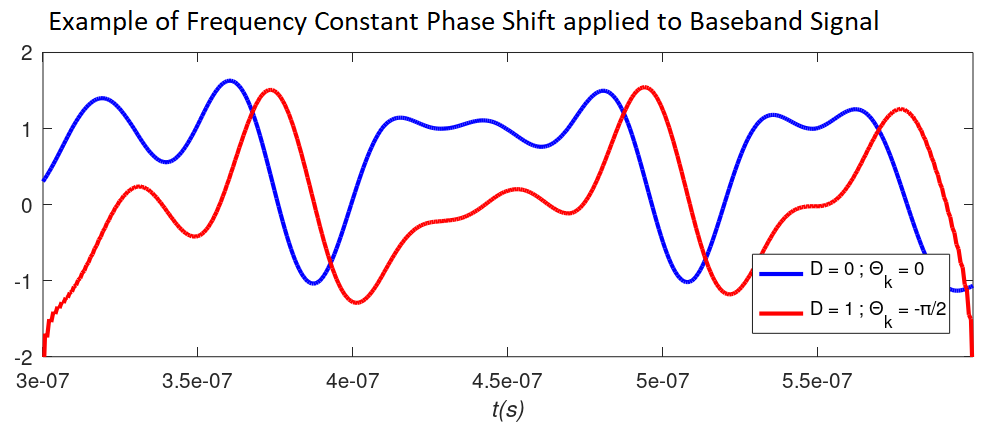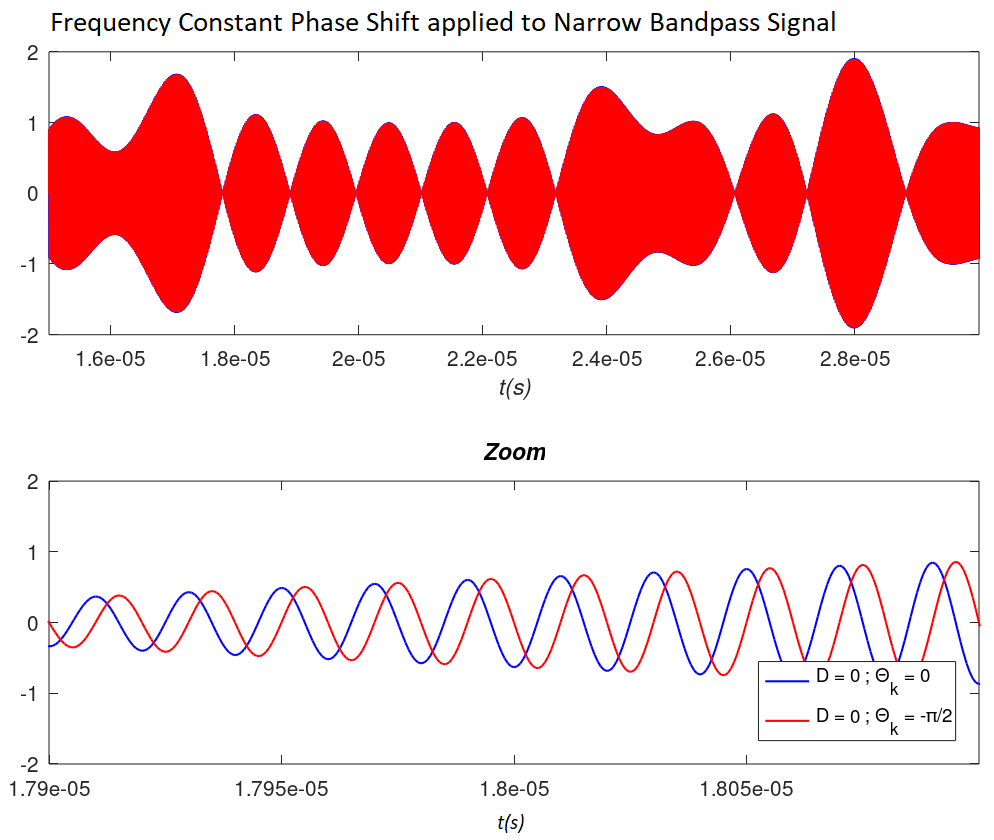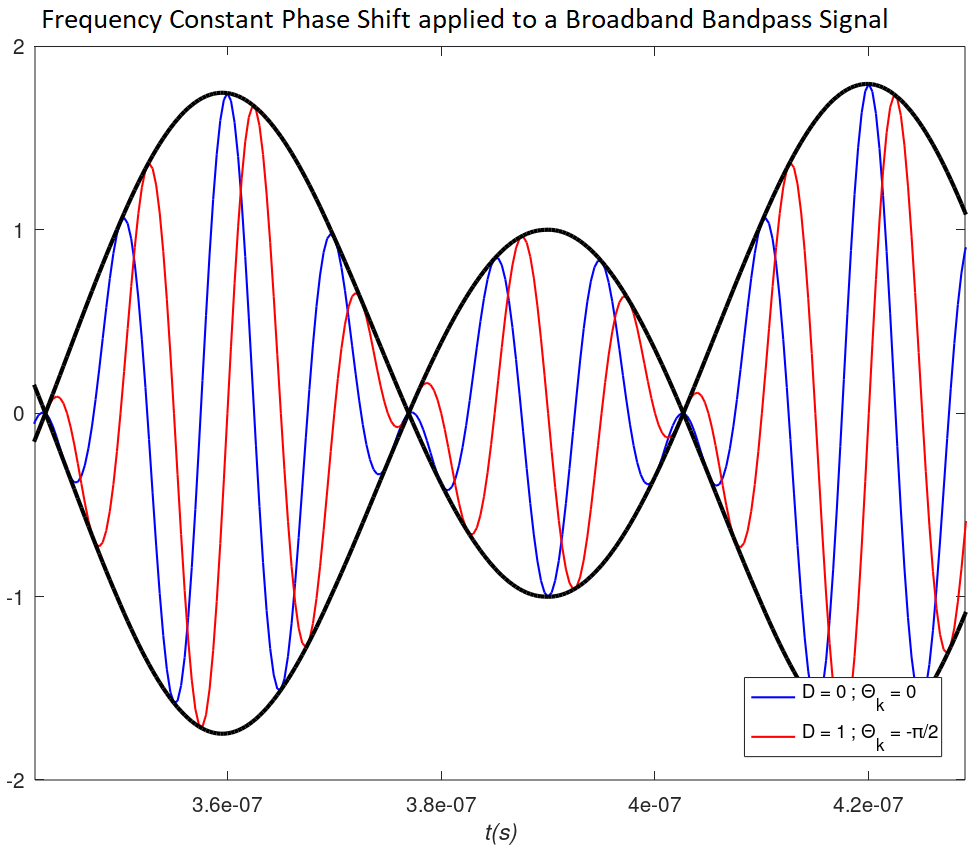Categories

# Frequency Constant Phase Shift and Distortion

Transmission media and electronic components can have multiple effects on the signals propagating through them  . To avoid phase distortion, it is necessary that the phase of the system, the phase shift introduced by the medium, is linear with frequency. This case, whose effect consists of a time delay of the signal, is often confused with the case of frequency constant phase shift.

As we will see in this article, constant phase shift, as opposed to linear phase shift, does result in distortion in the sense that the original signal changes its appearance. However, for the case of modulated carriers, the distortion produced by constant phase shift does not affect the signal envelope, coincident with the modulating signal. Therefore, in this particular case, the constant phase shift does not imply distortion in the demodulated signal.

Next, an analysis of the constant phase shift and its associated distortion for baseband and bandpass signals is performed.

## 1. Elements with Frequency Constant Phase Shift

This section introduces different elements and situations that give rise to constant phase shift. The classification is made discerning between electronic devices and transmission media.

### 1.1 Electronic Devices

In electronics there are many situations in which a component or processing generates constant phase shift. Sometimes constant phase shift is deliberately sought, while in other situations it is simply obtained collaterally without being the main objective. Listed below are cases and components that give rise to constant phase shift:

#### 1.1.1 Hilbert Transform

The Hilbert Transform  consists of applying a -90º phase shift to all the frequency components of a signal. In analog systems the Hilbert transform can be performed with one of the devices mentioned below. In digital systems the constant phase shift can be implemented by digital signal processing. The Hilbert transform is necessary to improve spectral efficiency by generating single sideband modulations.

#### 1.1.2 Hybrid Coupler

A Hybrid Coupler  is a device that divides the input power equally between two outputs. Its particularity is that the frequencies of both outputs have a constant phase shift between them, typically 90º or 180º. The most common are 90º (quadrature), and are designed to cover very wide bands in IQ mixers and single sideband modulators.

The aforementioned phase shift is evident in the S-parameter matrix of an ideal hybrid quadrature coupler:

\begin{equation} S= \frac{1}{\sqrt{\smash[b]{2}}} \begin{bmatrix} 0 & -j & -1 & 0 \\ -j & 0 & 0 & -1 \\ -1 & 0 & 0 & -j \\ 0 & -1 & -j & 0\end{bmatrix} \end{equation}

Assuming that the input is present at port 1, output 2 is phase shifted -90° while output 3 suffers a phase shift of -180°. This explains the 90° offset between the two outputs:

\begin{equation} \varphi= \phase{S_{21}}-\phase{S_{31}} = 90º \end{equation}

Note that the S-parameter matrix represents only one frequency. The particularity of these devices is that the 90° phase difference can be maintained for large bandwidths , becoming practically independent of frequency.

### 1.2 Transmission Media

The propagation of a signal through a transmission medium is articulated as a function of interrelated parameters such as phase velocity, group velocity or refractive index among others . If certain conditions are met, the medium can induce a frequency constant phase shift in the transmitted signal components.

We start from the following generic equation for f(x,t), a wave propagating at time t along the x-axis:

\begin{equation} f(x,t) = \cos[k(\omega) x-\omega t] \end{equation}

Where:

• \footnotesize \omega represents the angular frequency in rad/sg.
• \footnotesize k(\omega) represents the propagation constant in rad/m and is a function of \footnotesize \omega .

The transmitted signal typically consists of a sum of simultaneously propagating frequencies. Assuming that \footnotesize k(\omega) can be decomposed as follows:

\begin{equation} k(\omega) \approx a\omega + b \end{equation}

It follows that:

• b implies a constant and equal phase shift for all frequency components (bx).
• a is related to the propagation velocity and, consequently, the delay of the frequency components (ax).

This analysis is a simplification of reality, but it allows to introduce in a simple way the constant phase shift produced by a transmission medium. For a more rigorous and complex study, it is recommended to consult the propagation in an optical fiber .

## 2. Mathematical Model:Constant Phase Shift and Distortion

The analysis is performed in the context of linear and time invariant LTI systems . For simplicity, and in order to be able to quantify the distortion in a simple way, the study considers an input signal whose spectral density X(f) is shown in the following figure:

To ensure that all frequencies have the same power, Nyquist pulses are used to produce a uniform square spectrum. Such a spectrum is bounded by the initial frequency \footnotesize (f_o) and the final frequency \footnotesize (f_o+\Delta f) . Note that the baseband case would be obtained with \footnotesize f_o=0 .

### 2.1 Frequency Constant Phase Shift Transfer Function

The transfer function of a channel with no effect on amplitude, with delay \footnotesize t_g and constant phase shift \footnotesize \varTheta_k es:

\begin{equation} |H(f)| = 1\end{equation}

\begin{equation} \phase{H(f)} = -2{\pi}ft_g+\varTheta_k · sgn(f) \end{equation}

The function sgn(f) is equal to 1 for positive frequencies and -1 for negative frequencies. Often, even in reputable literature, the mistake is made of not including sgn(f) in the constant phase shift transfer function. The proof that this notation is correct is obtained by looking at the Fourier transform of a tone with generic frequency and phase shift :

\begin{equation} x(t) = \cos(2\pi f_ct+\phi) \end{equation}

\begin{equation} X(f) = \frac{1}{2}\left[e^{j\phi}\delta(f-f_c)+e^{-j\phi}\delta(f+f_c) \right] \end{equation}

For the case with no phase shift (\footnotesize \phi=0 ), the spectrum is real and consists of two Dirac deltas at the positive and negative frequencies. When a phase shift is applied to the tone (\footnotesize \phi\not=0 ), the phase shift at the positive frequency and the negation of the phase shift at the negative frequency appear. Explained in another way, the Fourier transform of a real signal has to be hermitic, and this is only possible by adding the function sgn(f) in the phase of the transfer function.

Therefore, ignoring the delay or frequency-linear phase shift produced by the component or medium, the spectrum of the output signal after applying the constant phase shift is the one observed in Y(f) in the previous figure. Since the phase is not linear with frequency, it follows that the signal will suffer distortion in the sense that its appearance changes. In other words, the output signal is not a delayed replica of the input signal. However, there is a qualification to this statement, as shown in the following section.

### 2.2 Constant Phase Shift on Modulated Carriers

Before quantifying the distortion produced by constant phase shift, a particular case is analyzed: modulated carriers. We start from a bandpass signal x(t) obtained from the components \footnotesize x_I(t) and \footnotesize x_Q(t) which modulate in phase and quadrature a carrier centered at \footnotesize \omega_c :

\begin{equation} x(t) = x_I(t)\cos(\omega_ct) - x_Q(t)\sin(\omega_ct)\end{equation}

The delay \footnotesize t_g and the constant phase shift \footnotesize \varTheta_k introduced in the transfer function (6) result in the following output signal :

\begin{equation} y(t) = x_I(t-t_g)\cos[\omega_c(t-t_g)+\varTheta_k] - x_Q(t-t_g)\sin[\omega_c(t-t_g)+\varTheta_k]\end{equation}

The above equation can be modified as follows:

\begin{equation} y(t) = x_I(t-t_g)\cos[\omega_c(t-t_d)] - x_Q(t-t_g)\sin[\omega_c(t-t_d)]\end{equation}

Where it is observed that \footnotesize t_g represents the group delay of the modulating signal while the phase delay of the carrier \footnotesize t_d is equal to:

\begin{equation}t_d = t_g - \frac{\varTheta_k}{\omega_c} \end{equation}

It is concluded that for modulated carriers, the transmission media introducing a constant phase shift give rise to different phase and group velocities. Comparing (9) and (11), it is observed that the overall signal is distorted due to this constant phase shift. However, the envelope or modulating signal remains unchanged, which allows it to be demodulated and recovered without distortion. Examples illustrating this effect are shown below.

In conclusion, when dealing with a bandpass channel or signal, the phase condition to avoid distortion can be relaxed. It has been shown that it is not necessary to require linear phase. Instead, the condition is that the group delay of the envelope \footnotesize t_g is constant according to the following equation:

\begin{equation} t_g = -\frac{1}{2\pi }\frac{d\phase{H(f)}}{df} \end{equation}

### 2.3 Constant Phase Shift Distortion

This section quantitatively models the distortion associated with the constant phase shift exerted on a signal. The author is unaware of previous publications that perform a similar exercise. This is probably because, in practice, it is difficult to quantify an effect that results in a change of “appearance”. Besides, real signals have frequency components of different amplitudes, which adds another level of complexity. That is why, for simplicity, this text uses the model described above assuming frequency components of the same amplitude to conform the signal.

#### 2.3.1 Two Tone Reduction

Due to the square spectrum, the constant phase shift distortion should be directly related to the delay of the furthest away tones: the initial frequency \footnotesize (f_o) and the final frequency \footnotesize (f_o+\Delta f) . Therefore, without loss of generality, the mathematical calculation can be reduced to the signal composed solely of both tones:

\begin{equation} s_i(t) = \cos(2\pi f_ot) + \cos[2\pi (f_o+\Delta f)t] \end{equation}

Once a frequency constant phase shift \footnotesize \varTheta_k is applied, it is obtained that:

\begin{equation} \begin{split} s_o(t) &= \cos(2\pi f_ot+\varTheta_k) + \cos[2\pi (f_o+\Delta f)t+\varTheta_k] \\ & \\&= \cos\left[2\pi f_o\left(t-\frac{-\varTheta_k}{2\pi f_o}\right)\right] + \cos\left[2\pi (f_o+\Delta f)\left(t-\frac{-\varTheta_k}{2\pi (f_o+\Delta f)}\right)\right] \end{split} \end{equation}

Consequently, both components undergo a different time delay \footnotesize \tau , which guarantees the change of aspect and linear phase distortion. Specifically:

\begin{equation} \tau(f_o) = \frac{-\varTheta_k}{2\pi f_o} \end{equation}

\begin{equation} \tau(f_o+\Delta f) = \frac{-\varTheta_k}{2\pi (f_o+\Delta f)} \end{equation}

Therefore, the delay difference between the two tones is equal to:

\begin{equation} \Delta\tau = \tau(f_o) - \tau(f_o+\Delta f) = \frac{-\varTheta_k}{2\pi}\frac{\Delta f}{f_o(f_o+\Delta f)} \end{equation}

Obviously, and in line with the previous equation, the delay difference grows with the signal bandwidth. In such case its value tends to:

\begin{equation} \Delta\tau\Bigr|_{\substack{ \\ \\ \Delta f >> f_o}} \approx \frac{-\varTheta_k}{2\pi f_o} \end{equation}

#### 2.3.2 Distortion Quantification

The objective is to give a value to the distortion D that allows quantitative evaluation and comparison of the distortion associated with different situations. For this purpose, it is necessary to identify the worst possible case of \footnotesize \Delta\tau from the distortion point of view. In that sense, evaluating equation (15), it can be deduced that:

• Values of \footnotesize \varTheta_k equal to multiples of \footnotesize \pm 2\pi do not produce any distortion, since they reproduce the original signal.
• Values of \footnotesize \varTheta_k equal to odd multiples of \footnotesize \pm \pi produce inversion. In general, inversion is not considered to be distortion, since it only changes the sign of the original signal.
• Consequently, the most unfavorable case of distortion occurs when \footnotesize \varTheta_k is equal to odd multiples of \footnotesize \pm \pi/2 . Hence, from now on we consider a \footnotesize \varTheta_k' equal to the phase \footnotesize \varTheta_k wrapped in the range \footnotesize [-\pi/2,+\pi/2] . For convenience, the discussion is continued by employing \footnotesize \varTheta_k without quotation marks, which should not cause confusion.

In line with the above reasoning, we define the worst case of \footnotesize \Delta\tau from the distortion point of view as:

\begin{equation} \Delta \tau_{max} = \Delta\tau\Bigr|_{\substack{ \\ \\ \Delta f >> f_o \\ \\ \varTheta_k=\mp \pi/2}} \approx \pm \frac{1}{4f_o} \end{equation}

Finally, from equations (18) and (20), we define the distortion D associated with the constant phase shift \footnotesize \varTheta_k exerted on the signal as:

\begin{equation} D = \frac{|\Delta\tau|}{|\Delta\tau|_{max}} = \frac{2|\varTheta_k|}{\pi}\frac{\Delta f}{f_o+\Delta f} \end{equation}

Thus the D value is delimited between 0 and 1, with 1 representing the worst case of distortion. In this way, the distortion produced by the constant phase shift on the signal can be quantified, as illustrated in the following section.

## 3. Effects of Constant Phase Shift

Below they are discussed in more detail and examples are provided for all possible cases divided into: baseband signals and narrowband and broadband bandpass signals.

### 3.1 Baseband Signals

The distortion obtained in a baseband signal can be obtained from equation (21) by making \footnotesize f_o tend to zero (\footnotesize f_o \to 0 ):

\begin{equation} D_{baseband} = D\Bigr|_{f_o \to 0}\approx \frac{2|\varTheta_k|}{\pi} \end{equation}

It is observed that the distortion depends only on the constant phase shift and is independent of the bandwidth \footnotesize \Delta f . This is due to the high period of the lowest frequency component, which tends to maximize the delay difference between the frequency components. As reasoned above, the worst case distortion occurs for the Hilbert Transform, \footnotesize \varTheta_k = -\pi/2. The following plot shows the distortion associated with this constant phase shift in a baseband signal:Hilbert transform (red) applied on a baseband signal (blue) of \footnotesize \Delta f = 50 MHz generated with Nyquist pulses. It allows observing the maximum distortion associated with a constant phase shift.

### 3.2 Narrowband Bandpass Signals

Following the notation of the presented model, the narrowband bandpass signals are characterized by the following condition: \footnotesize \Delta f << f_o . Therefore, mathematically, from equation (21) it follows:

\begin{equation} D_{narrowband} = D\Bigr|_{\Delta f << f_o} \approx 0 \end{equation}

Obviously, the distortion tends to zero, since the delay difference between the different tones that compose the signal is very small or even negligible. This effect can be seen in the following graph, where the worst case constant phase shift (\footnotesize \varTheta_k=-\pi/2) has been applied to a narrow bandpass signal:Original narrowband signal (blue) generated with \footnotesize f_o =49 MHz and \footnotesize \Delta f =2 MHz. Its Hilbert Transform in red. The top plot shows the complete signals, while the bottom plot shows a zoom.

Unnoticeable even with the graph zoomed in, the constant phase shift produces a very small distortion. In particular, the peaks of the red signal are slightly different from the contiguous peaks of the blue signal. In practical terms, the constant phase shift has been translated into a constant delay for all frequency components. Consequently, the appearance of the original signal hardly changes. In any case, the signal envelope remains unchanged.

Finally, the case of broadband bandpass signals is analyzed. In this case it is satisfied that \footnotesize \Delta f >> f_o . Applying this condition, from equation (21), the distortion obtained is:

\begin{equation} D_{broadband} = D\Bigr|_{\Delta f >> f_o}\approx \frac{2|\varTheta_k|}{\pi} \end{equation}

For this case the distortion tends to depend only on the constant phase shift value, being maximum for \footnotesize \varTheta_k=\pm \pi/2. Due to the wide bandwidth of the signal, the delay difference between the different frequency components tends to be maximum. The following graph shows an example where the distortion is clearly observed:Broadband bandpass signal with \footnotesize \Delta f =200 MHz (blue). Hilbert transform of the blue signal (red). Envelope or modulating signal (black).

The original signal (blue) and the phase shifted signal (red) are clearly different, showing that the constant phase shift has produced distortion. However, and in line with the demonstration in section 2.2, the envelope or modulating signal (black) remains unchanged. Therefore, distortion due to frequency constant phase shift in a bandpass signal does not necessarily translate into distortion in the transmission system.